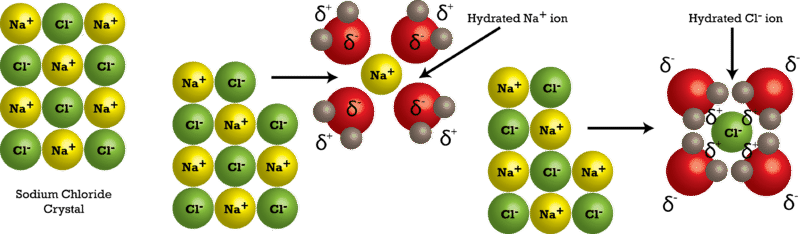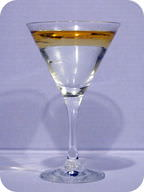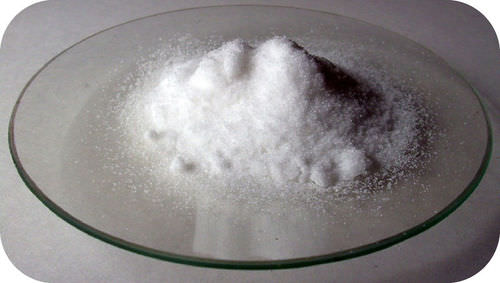# 3.3: The Dissolving Process

$$\newcommand{\vecs}{\overset { \rightharpoonup} {\mathbf{#1}} }$$ $$\newcommand{\vecd}{\overset{-\!-\!\rightharpoonup}{\vphantom{a}\smash {#1}}}$$$$\newcommand{\id}{\mathrm{id}}$$ $$\newcommand{\Span}{\mathrm{span}}$$ $$\newcommand{\kernel}{\mathrm{null}\,}$$ $$\newcommand{\range}{\mathrm{range}\,}$$ $$\newcommand{\RealPart}{\mathrm{Re}}$$ $$\newcommand{\ImaginaryPart}{\mathrm{Im}}$$ $$\newcommand{\Argument}{\mathrm{Arg}}$$ $$\newcommand{\norm}{\| #1 \|}$$ $$\newcommand{\inner}{\langle #1, #2 \rangle}$$ $$\newcommand{\Span}{\mathrm{span}}$$ $$\newcommand{\id}{\mathrm{id}}$$ $$\newcommand{\Span}{\mathrm{span}}$$ $$\newcommand{\kernel}{\mathrm{null}\,}$$ $$\newcommand{\range}{\mathrm{range}\,}$$ $$\newcommand{\RealPart}{\mathrm{Re}}$$ $$\newcommand{\ImaginaryPart}{\mathrm{Im}}$$ $$\newcommand{\Argument}{\mathrm{Arg}}$$ $$\newcommand{\norm}{\| #1 \|}$$ $$\newcommand{\inner}{\langle #1, #2 \rangle}$$ $$\newcommand{\Span}{\mathrm{span}}$$

Learning Outcomes

• Define a solution and describe the parts of a solution.
• Describe how an aqueous solution is formed from both ionic compounds and molecular compounds.
• Recognize that some compounds are insoluble in water.
• Describe the differences among strong electrolytes, weak electrolytes, and nonelectrolytes.

## Forming a Solution

When one substance dissolves into another, a solution is formed. A solution is a homogenous mixture consisting of a solute dissolved into a solvent. The solute is the substance that is being dissolved, while the solvent is the dissolving medium. Solutions can be formed with many different types and forms of solutes and solvents. In this chapter, we will focus on solution where the solvent is water. An aqueous solution is water that contains one or more dissolved substance. The dissolved substances in an aqueous solution may be solids, gases, or other liquids.

In order to be a true solution, a mixture must be stable. When sugar is fully dissolved into water, it can stand for an indefinite amount of time, and the sugar will not settle out of the solution. Further, if the sugar-water solution is passed through a filter, it will remain with the water. This is because the dissolved particles in a solution are very small, usually less than $$1 \: \text{nm}$$ in diameter. Solute particles can be atoms, ions, or molecules, depending on the type of substance that has been dissolved.Figure $$\PageIndex{1}$$: When a colored solution is passed through a filter, the entire solution, both solute and solvent, pass through unchanged.

### The Dissolving Process

Water typically dissolves most ionic compounds and polar molecules. Nonpolar molecules, such as those found in grease or oil, do not dissolve in water. We will first examine the process that occurs when an ionic compound, such as table salt (sodium chloride), dissolves in water.

Water molecules move about continuously due to their kinetic energy. When a crystal of sodium chloride is placed into water, the water's molecules collide with the crystal lattice. Recall that the crystal lattice is composed of alternating positive and negative ions. Water is attracted to the sodium chloride crystal because water is polar; it has both a positive and a negative end. The positively charged sodium ions in the crystal attract the oxygen end of the water molecules because they are partially negative. The negatively charged chloride ions in the crystal attract the hydrogen end of the water molecules because they are partially positive. The action of the polar water molecules takes the crystal lattice apart (see figure below).Figure $$\PageIndex{2}$$: The process of an ionic sodium chloride crystal being dissolved and hydrated by water.

After coming apart from the crystal, the individual ions are then surrounded by solvent particles in a process called solvation. Note in the figure above that the individual $$\ce{Na^+}$$ ions are surrounded by water molecules with the oxygen atom oriented near the positive ion. Likewise, the chloride ions are surrounded by water molecules with the opposite orientation. Hydration is the process of solute particles being surrounded by water molecules arranged in a specific manner. Hydration helps to stabilize aqueous solutions by preventing the positive and negative ions from coming back together and forming a precipitate.

Table sugar is made of the molecular compound sucrose $$\left( \ce{C_{12}H_{22}O_{11}} \right)$$. Solid sugar consists of individual sugar molecules held together by intermolecular attractive forces. When water dissolves sugar, it separates the individual sugar molecules by disrupting the attractive forces, but it does not break the covalent bonds between the carbon, hydrogen, and oxygen atoms. Dissolved sugar molecules are also hydrated. The hydration shell around a molecule of sucrose is arranged so that its partially negative oxygen atoms are near the partially positive hydrogen atoms in the solvent, and vice versa.

### Insoluble Compounds

Not all compounds dissolve well in water. Some ionic compounds, such as calcium carbonate $$\left( \ce{CaCO_3} \right)$$ and silver chloride $$\left( \ce{AgCl} \right)$$, are nearly insoluble. This is because the attractions between the ions in the crystal lattice are stronger than the attraction that the water molecules have for the ions. As a result, the crystal remains intact. The solubility of ionic compounds can be predicted using the solubility rules as shown in Table $$\PageIndex{1}$$.

Table $$\PageIndex{1}$$: Solubility rules for ionic compounds in water.Nonpolar compounds also do not dissolve in water. The attractive forces that operate between the particles in a nonpolar compound are weak dispersion forces. In order for a nonpolar molecule to dissolve in water, it would need to break up some of the hydrogen bonds between adjacent water molecules. In the case of an ionic substance, these favorable interactions are replaced by other attractive interactions between the ions and the partial charges on water. However, interactions between nonpolar molecules and water are less favorable than the interactions that water makes with itself. When a nonpolar liquid such as oil is mixed with water, two separate layers form, because the liquids will not dissolve into each other (see figure below). When a polar liquid like ethanol is mixed with water, they completely blend and dissolve into one another. Liquids that dissolve in one another in all proportions are said to be miscible. Liquids that do not dissolve in one another are called immiscible. The general rule for deciding if one substance is capable of dissolving another is "like dissolves like", where the property being compared is the overall polarity of the substance. For example, a nonpolar solid such as iodine will dissolve in nonpolar lighter fluid, but it will not dissolve in polar water.Figure $$\PageIndex{3}$$: Water and oil form separate layers when they are mixed because the nonpolar oil will not dissolve into the polar water. The oil forms the top layer because it is less dense than water.

## Electrolytes and Nonelectrolytes

An electrolyte is a compound that conducts an electric current when it is dissolved in water or melted. In order to conduct a current, a substance must contain mobile ions that can move from one electrode to the other. All ionic compounds are electrolytes. When ionic compounds dissolve, they break apart into ions, which are then able to conduct a current. Even insoluble ionic compounds, such as $$\ce{CaCO_3}$$, are considered electrolytes because they can conduct a current in the molten (melted) state.Figure $$\PageIndex{4}$$: An apparatus for testing the conductivity of a solution.

A nonelectrolyte is a compound that does not conduct an electric current in either aqueous solution or in the molten state. Many molecular compounds, such a sugar or ethanol, are nonelectrolytes. When these compounds dissolve in water, they do not produce ions. Illustrated below is the difference between an electrolyte and a nonelectrolyte.

### Dissociation

Earlier, you saw how an ionic crystal lattice breaks apart when it is dissolved in water. Dissociation is the separation of ions that occurs when a solid ionic compound dissolves. Simply undo the crisscross method that you learned when writing chemical formulas for ionic compounds, and you are left with the components of an ionic dissociation equation. The subscripts for the ions in the chemical formulas become the coefficients of the respective ions on the product side of the equations. Shown below are dissociation equations for $$\ce{NaCl}$$, $$\ce{Ca(NO_3)_2}$$, and $$\ce{(NH_4)_3PO_4}$$.

\begin{align} &\ce{NaCl} \left( s \right) \rightarrow \ce{Na^+} \left( aq \right) + \ce{Cl^-} \left( aq \right) \\ &\ce{Ca(NO_3)_2} \left( s \right) \rightarrow \ce{Ca^{2+}} \left( aq \right) + 2 \ce{NO_3^-} \left( aq \right) \\ &\ce{(NH_4)_3PO_4} \left( s \right) \rightarrow 3 \ce{NH_4^+} \left( aq \right) + \ce{PO_4^{3-}} \left( aq \right) \end{align}

One formula unit of sodium chloride dissociates into one sodium ion and one chloride ion. The calcium nitrate formula unit dissociates into one calcium ion and two nitrate ions, because the $$2+$$ charge of each calcium ion requires two nitrate ions (each with a charge of $$1-$$) to form an electrically neutral compound. The ammonium phosphate formula unit dissociates into three ammonium ions and one phosphate ion.

Do not confuse the subscripts of the atoms within the polyatomic ion for the subscripts that result from the crisscrossing of the charges that make the original compound neutral. The 3 subscript of the ntirate ion and the 4 subscript of the ammonium ion are part of the polyatomic ion and remain a part of the ionic formula after the compound dissociates. Notice that the compounds are solids $$\left( s \right)$$ that become ions when dissolved in water, producing an aqueous solution $$\left( aq \right)$$.Figure $$\PageIndex{5}$$: Calcium nitrate is a typical ionic compound. In an aqueous solution, it dissociates into calcium ions and nitrate ions.

Nonelectrolytes do not dissociate when forming an aqueous solution. An equation can still be written that simply shows the solid going into solution. For example, the process of dissolving sucrose in water can be written as follows:

$\ce{C_{12}H_{22}O_{11}} \left( s \right) \rightarrow \ce{C_{12}H_{22}O_{11}} \left( aq \right)$

### Strong and Weak Electrolytes

Some polar molecular compounds are nonelectrolytes when the are in their pure state but become electrolytes when they are dissolved in water. Hydrogen chloride $$\left( \ce{HCl} \right)$$ is a gas in its pure molecular state and is a nonelectrolyte. However, when $$\ce{HCl}$$ is dissolved in water, it conducts a current well because the $$\ce{HCl}$$ molecule ionizes into hydrogen and chloride ions.

$\ce{HCl} \left( g \right) \rightarrow \ce{H^+} \left( aq \right) + \ce{Cl^-} \left( aq \right)$

When $$\ce{HCl}$$ is dissolved into water, it is called hydrochloric acid. Ionic compounds and some polar compounds are completely broken apart into ions and thus conduct a current very well. A strong electrolyte is a solution in which almost all of the dissolved solute exists as ions.

Some other polar molecular compounds become electrolytes upon being dissolved into water but do not ionize to a very great extent. For example, nitrous acid $$\left( \ce{HNO_2} \right)$$ only partially ionizes into hydrogen ions and nitrite ions when dissolved in water. Aqueous nitrous acid is composed of only about $$5\%$$ ions and $$95\%$$ intact nitrous acid molecules A weak electrolyte is a solution in which only a small fraction of the dissolved solute exists as ions. The equation showing the ionization of a weak electrolyte utilizes an equilibrium arrow, indicating an equilibrium between the reactants and products.

$\ce{HNO_2} \left( aq \right) \rightleftharpoons \ce{H^+} \left( aq \right) + \ce{NO_2^-} \left( aq \right)$